# High School Math : Radicals

## Example Questions

1 2 3 5 Next →

### Example Question #85 : Algebra Ii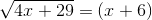No real solutionsExplanation:

Begin by squaring both sides of the equation: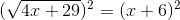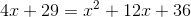Now, combine like terms: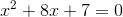Factor the equation: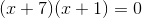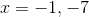However, when plugging in the values,does not work. Therefore, there is only one solution: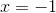### Example Question #86 : Algebra Ii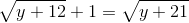Explanation:

Begin by squaring both sides of the equation: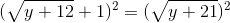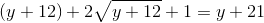Now, combine like terms and simplify: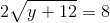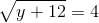Once again, take the square of both sides of the equation: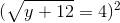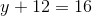Solve the linear equation: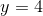### Example Question #1 : Solving Radical Equations And Inequalities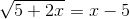Explanation:

Begin by taking the square of both sides: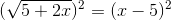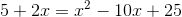Combine like terms: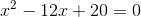Factor the equation and solve: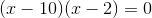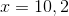However, when plugging in the values,does not work. Therefore, there is only one solution: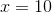### Example Question #2 : Solving Radical Equations And Inequalities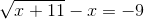Explanation:

To solve the radical expression, begin by subtractingfrom each side of the equation: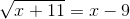Now, square both sides of the equation: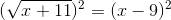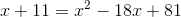Combine like terms: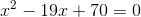Factor the expression and solve: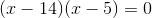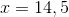However, when plugged into the original equation,does not work because the radical cannot be negative. Therefore, there is only one solution: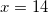### Example Question #3 : Solving Radical Equations And Inequalities

Solve the equation for.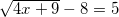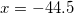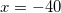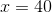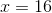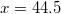Explanation:Addto both sides.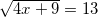Square both sides.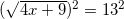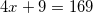Isolate.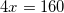### Example Question #4 : Solving Radical Equations And Inequalities

Solve for: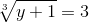Explanation:

Begin by cubing both sides: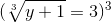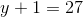Now we can easily solve: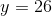1 2 3 5 Next →